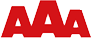## One die probabilities

A standard die has six sides with the values one to six. For a single roll of a fair die, the probability of rolling each value is exactly 1/6. A die doesn’t have a memory, so even if you haven’t rolled a six in hundreds of attempts, it still has the same probability as any other value.

Visualizing probability on the web is easy using SpreadsheetConverter and it’s powerful support for live charts. In this example we use a bar chart to visualize the outcome of a single die being rolled twenty times.

Test this calculator in other formats:

## Two dice probabilities

When you roll two dice simultaneously, the total of both rolls is not evenly distributed. The likelyhood of getting a sum of 7 is 1/6, more than any other value. The likelyhood of getting a sum of 2 or 12 is only 1/36.

To test if this could qualify as one of our profitable bar tricks, we created a two dice simulator, that lets you roll two dice twenty times and study the outcome in a column chart.

Test this calculator in other formats

## Try this Excel add-in now!Click on Download to install and test this Excel add-in for Windows.